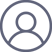张伟2021-4-22 15:31

（1）理解拉格朗日乘子法；（2）为什么拉格朗日乘子法构造的公式又可写为min max f(x).

（一）理解拉格朗日乘子法2.等高线3 拉格朗日乘子法

3.1 求解3.2 定义意思是subject to，服从于，约束于的意思。3.3 变形3.4 多个约束条件往更高维度走的话，多约束条件的情况下，问题变为了围成的曲线和 相切，直观上看必然在张成的空间中：（二）min max f(x)

KKT条件是满足强对偶条件的优化问题的必要条件，可以这样理解：我们要求min f(x),  且满足约束条件

s.t.  g_i(x) <= 0; i =1, ..., n

h_j(x) = 0; j =1, ..., m

我们构造了函数L(a, b, x) = f(x) + a*g(x) + b*h(x)，a>=0，

此处详细解释为什么在KKT条件下就可以将f(x)写为：max_{a,b} L(a,b,x)：我们的目标函数是要求min f(x)，为此我们构造了函数L(a, b, x) = f(x) + a*g(x) + b*h(x)（a>=0），那么，由于约束条件为h(x)=0, g(x)<=0，所以我们这里为了使f(x)与L(a, b, x) 等价，用了两个方法：一是给取L(a,b,x)的最大值，这样便于后面用求导方法计算最优值，二是要满足KKT条件，因为a*g(x)是<=0，所以L(a,b,x)只有在a*g(x) = 0的情况下才能取得最大值，否则，就不满足约束条件，因此max_{a,b} L(a,b,x)在满足约束条件的情况下就是f(x)。

因此我们的目标函数minf(x)可以写为 min_x max_{a,b} L(a,b,x)。如果用对偶表达式：

max_{a,b} min_x  L(a,b,x)；

由于我们的优化是满足强对偶的（强对偶就是说对偶式子的最优值是等于原问题的最优值的），所以在取得最优值x0的条件下，它满足 f(x0) = max_{a,b} min_x  L(a,b,x) = min_x max_{a,b} L(a,b,x) =f(x0)，我们来看看中间两个式子发生了什么事情：

f(x0) = max_{a,b} min_x  L(a,b,x) =  max_{a,b} min_x f(x) + a*g(x) + b*h(x) =  max_{a,b}

f(x0)+a*g(x0)+b*h(x0) = f(x0)

可以看到上述加黑的地方本质上是说 min_x f(x) + a*g(x) + b*h(x) 在x0取得了最小值，用fermat定理，即是说对于函数 f(x) + a*g(x) + b*h(x)，求取导数要等于零，即

f(x)的梯度+a*g(x)的梯度+ b*h(x)的梯度 = 0

这就是kkt条件中第一个条件：L(a, b, x)对x求导为零。

【参考】

https://www.zhihu.com/question/38586401

https://blog.csdn.net/weixin_41694823/article/details/83411435